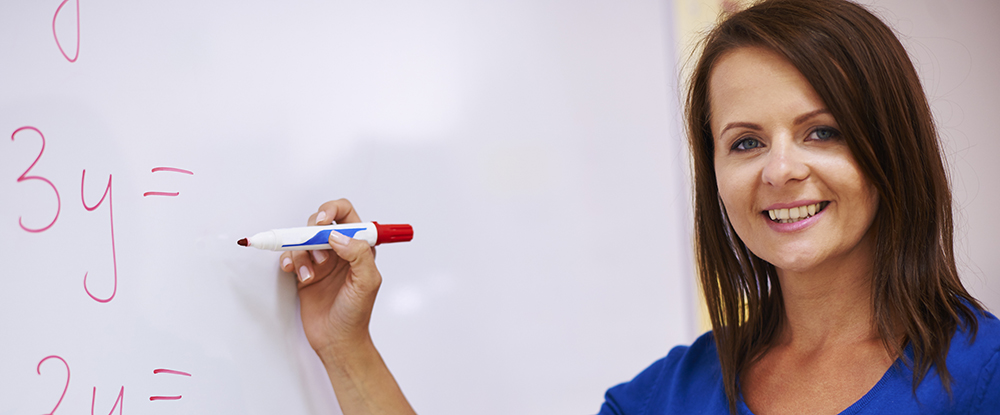# Degree Info

## Mathematics - Secondary Education Concentration

You will develop strong skills in logic and critical thinking through this program. Gain a mastery of calculus, algebra, geometry, statistics, and computer programming. Learn how mathematics is a critical tool for technological advancement and practical problem-solving in a complex natural and social world.

This degree program was developed for those who want to teach at the secondary level. You will gain hands-on, in-classroom experiences. Prospective secondary education teachers learn how to identify and be sensitive to the unique needs, challenges and learning styles of diverse student populations.

### Education 6-12 Licensure Courses

Required Courses

• The Teaching Profession I
• The Exceptional Child
• Educational Psychology
• Foundations of Schools in a Diverse Society
• Secondary Methods and RICA
• Specialized Methods/Practicum
• SEI Methods for ELL II
• Secondary Student Teaching I
• Secondary Student Teaching II

#### Mathematics Major Courses

Discrete Mathematics |
Discrete Mathematics is an introductory course into mathematical structures that are primarily discrete as opposed to continuous. Such topics to be covered include logic, relations, basic set theory, graph theory, languages, and finite state machines.

Calculus I |
Studies basic notions of a derivative and integral with basic techniques and applications to elementary functions. Emphasis on intuitive understanding and theorem application. Includes computer laboratory component.

Calculus II |
Study of integration techniques of infinite series and applications of derivatives and integrals to a wide variety of geometric, physical and behavioral problems. Includes computer laboratory component.

Linear Algebra |
Introduces algebra and geometry of vectors, matrices and linear transformations. Designed for all students using simultaneous equations and matrices. Also introduces reading and writing rigorous mathematical proofs.

Transition to Higher Mathematics |
Emphasizes the enhancement of the student’s ability to write and comprehend mathematical proof. The course takes a rigorous look at standard methods of proof and their validity.

Calculus III |
Extension of study of differentiation and integration to vector function and functions of several variables.
Emphasis on intuitive understanding of concepts and on applications. Includes computer laboratory
component.

Mathematical Statistics I |
Axioms and theorems of elementary probability, random variables, probability distributions, expectation, mean, variance, moment generating functions of probability distributions, multivariate distributions, and the central limit theorem. Designed to prepare student to take actuarial exam in probability and statistics. Intended for mathematics majors.

Mathematical Statistics II |
Mathematical Statistics II is the second half of a solid undergraduate treatment of statistical theory. Emphasis is placed on inferential statistics. Topics to be covered include survey sampling, descriptive statistics, parameter estimation, hypothesis testing, two-sample issues, analysis of variance, and analysis of categorical data.

Differential Equations |
Topics include various techniques for finding solutions of differential equations in one variable, general characteristics of solutions of first and second-order equations, boundary value problems, series solution techniques, and systems of linear equations. Studies historical development of the subject and applications to problems in sciences.

Abstract Algebra |
Study of elementary number theory, groups, rings, and fields. Includes induction, fundamental theorem of arithmetic, congruence relations, isomorphism theorems, and quotient structures. Culminates with survey of Galois Theory.

Numerical Methods |
Introduces numerical techniques and algorithms fundamental to scientific computer work including discussion of error, roots of equations, interpolation, systems of equations, numerical integration and methods of solution of ordinary differential equations.

Introduction to Real Analysis |
Study of real number system and its application to limit concept. Includes proofs of basic theorems on derivatives, integrals and continuity. Emphasis on rigor.

Integrative Seminar in Mathematics |
Capstone course that guides student in development of an integrative project that demonstrates achievement of learning outcomes in the mathematics major.

Full Degree Catalog